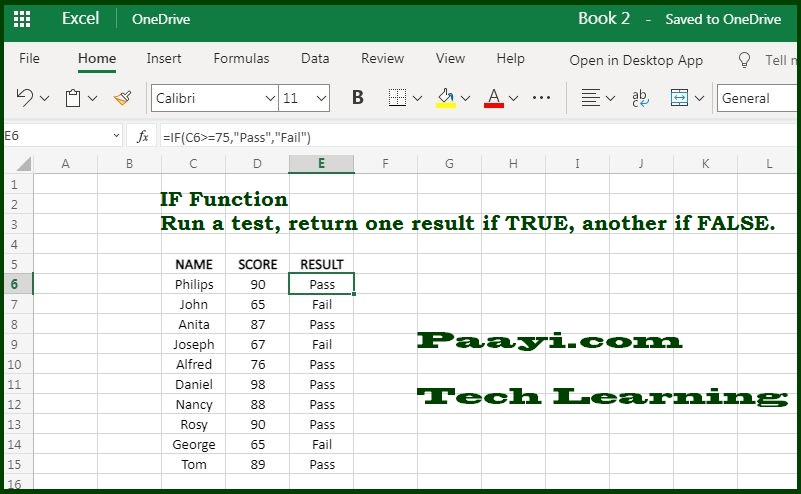# Learn How to Use Microsoft Excel IF Function Test Specific Condition

Written by | 0 Comments | 876 Views

In this article, you will learn how to use the Microsoft Excel IF function and its prime function in Microsoft Excel. You will also get to know the Microsoft Excel IF function return value and syntax with the help of some examples.

Microsoft Excel IF Function Test Specific Condition

The purpose of the Microsoft Excel IF function is to test a specific condition. The IF function is used to run a logical test and returns a particular value for a TRUE result and another for a FALSE result.

For example, to "pass" scores above 80: =IF(A1>80,"Pass","Fail"). You can easily test more than one condition by nesting IF functions. The IF function can be combined with logical functions like "AND" and "OR" for enhancing the logical test.

Return Value of IF Function

The values TRUE or FALSE, supplied by you

Syntax of IF Function

=IF (Logical-Test, [Value-If-True], [Value-If-False])

• Logical-Test: A value or logical expression which is evaluated as TRUE or FALSE.
• Value-If-True: (Optional)The value returns TRUE when the logical test evaluates to be true.
• Value-If-False: (Optional) The value returns FALSE when the logical test evaluates to be false.

How to Use Microsoft Excel IF Function?The IF function is used to run the logical test, and it evaluates differently as per the result TRUE or FALSE. The first argument, Logical-Test, is an expression that returns the value either TRUE or FALSE. The Value-If-True and Value-If-False are optional, but you must provide at least one of them. The result from the IF function can be a value, a cell reference, or another formula.

IF Function Examples

• =IF(A1<100,A1 * 6%,A1 * 8%)

The above formula will return A1 * 6% when A1 is less than 100 and when A1 is greater than or equal to 100, it will return A1 * 8%.

• =IF(A1<75, "F", IF (A1<80, "D", IF(A1<85, "C", IF(A1<90, "B", "A"))))

You can also utilize the IF function to provide the grade rather than just PASS or FAIL, as given in the example above.